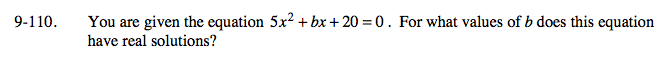Home > A2C > Chapter 9 > Lesson 9.2.3 > Problem9-110

9-110.

You are given the equation 5x2 + bx + 20 = 0. For what values of b does this equation have real solutions? Homework Help ✎Use the Quadratic Formula.

$x=\frac{-b\pm\sqrt{b^{2}-4(5)(20)}}{2(5)}$

The equation will have real solutions as long as the value under the radical is greater than or equal to zero.